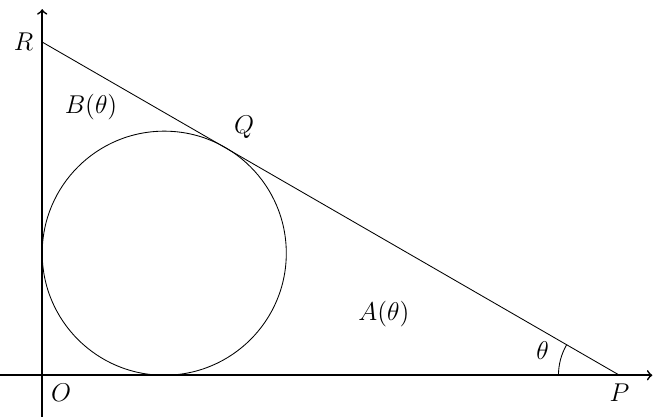###### back to index | new

Let $r$ and $s$ be integers. Find the condition such that the expression $\frac{6^{r+s}\times 12^{r-s}}{8^r\times 9^{r+2s}}$ is an integer.

Find the number of solutions to the equation $7\sin x + 2\cos^2 x = 5$ for $0\le x < 2\pi$.

Find the point on the circle $(x − 5)^2 + (y − 4)^2 = 4$ which is closest to the circle $(x − 1)^2 + (y − 1)^2 = 1$.

Find the number of real number solutions to the equation: $8^x +4=4^x + 2^{x+2}$.

Let function $f(x)$ satisfy:  $$\int^1_0 3f (x) dx +\int^2_1 2f (x) dx = 7$$

and $$\int^2_0 f (x) dx + \int^2_1 f (x) dx = 1$$

Find the value of $$\int^2_0 f (x) dx$$

Let $f_n (x) = (2 + (−2)^n ) x^2 + (n + 3) x + n^2$.

1. Write down $f_3(x)$ and find its maximum value. Also determine for what value of $n$ does the function $f_n(x)$ have a maximum value (as $x$ varies). You do not need to compute this maximum value.
2. Write down $f_1(x)$. Calculate $f_1(f_1(x))$ and $f_1(f_1(f_1(x)))$. Find an expression, simplified as much as possible, for $$\underbrace{f_1(f_1(\cdots f_1(x)))}_{k}$$
3. Write down $f_2(x)$. Find the degree of the function $$\underbrace{f_2(f_2(\cdots f_2(x)))}_{k}$$

Let $f(c)=\int_0^1\left( (x-c)^2 + c^2\right)dx$ where $c$ is a real number. Find the minimal value of $f(c)$ as $c$ varies and the maximum value of $f(\sin\theta)$ as $\theta$ varies.

In the diagram below, a line is tangent to a unit circle centered at $Q (1, 1)$ and intersects the two axes at $P$ and $R$, respectively. The angle $\angle{OPR}=\theta$. The area bounded by the circle and the $x-$axis is $A(\theta)$ and the are bounded by the circle and the $y-$axis is $B(\theta)$.

1. Show the coordinates of the point $Q$ is $(1+\sin\theta, 1+\cos\theta)$. Find the equation of line $PQR$ and determine the coordinates of $P$.
2. Explain why $A(\theta)=B\left(\frac{\pi}{2}-\theta\right)$ always holds and calculates $A\left(\frac{\pi}{2}\right)$.
3. Show that $A\left(\frac{\pi}{3}\right)=\sqrt{3}-\frac{\pi}{3}$.Find the area of the region bounded by the curve $y=\sqrt{x}$, the line line $y=x-2$, and the $x-$ axis.

Find the number of $k$ such that the function $y=e^{kx}$ satisfies the equation $$\left(\frac{d^2y}{dx^2}+\frac{dy}{dx}\right)\left(\frac{dy}{dx}-y\right)=y\frac{dy}{dx}$$

Let $a$, $b$, $c$ and $d$ be real numbers. Find the relation of these four numbers such that the two curves $y=ax^2+c$ and $y=bx^2 + d$ have exactly two points of intersections.

A circle of radius $2$, center on the origin, is drawn on a grid of points with integer coordinates. Let $n$ be the grid points that lie within or on the circle. What is the smallest amount of radius needs to increase by for there to be $(2n-5)$ grid points within or on the circle?

A particle moves in the $xy$-plane, starting at the origin $(0, 0)$. At each turn, the particle may move in one of the two ways:

• it may move two to the right and one up
• it may move one to the right and two up

What is the closet distance the particle may come to the point $(25, 75)$?

Find the value of $c$ such that two parabolas $y=x^2+c$ and $y^2=x$ touch at a single point.